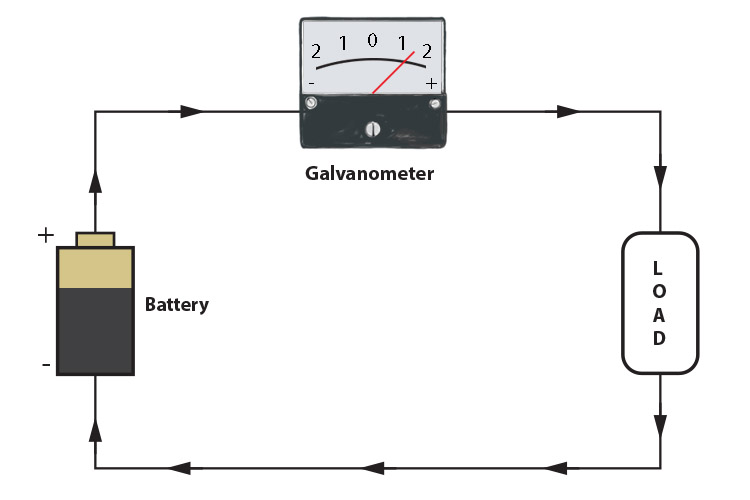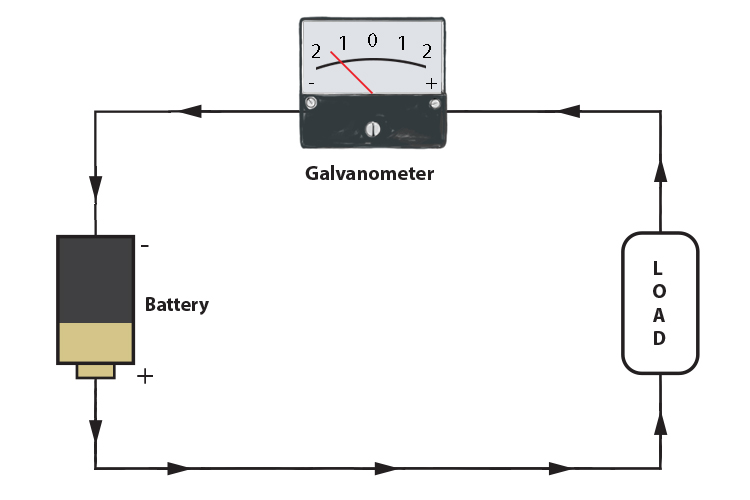# The difference between an ammeter and galvanometer

Before we can discuss Fleming's right-hand rule we need to understand the difference between an ammeter and a galvanometer.

## Ammeter

An ammeter is a measuring instrument used to measure the magnitude of the current in a circuit. Electric currents are measured in amperes, hence the name ammeter.## Galvanometer

A galvanometer measures both the direction of the current and its magnitude.Galvanometer shows 1.5 amps positive.Galvanometer shows 1.5 amps negative.